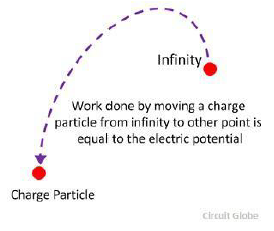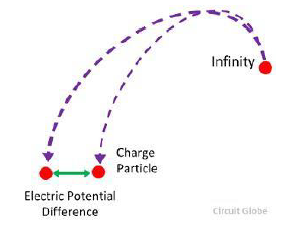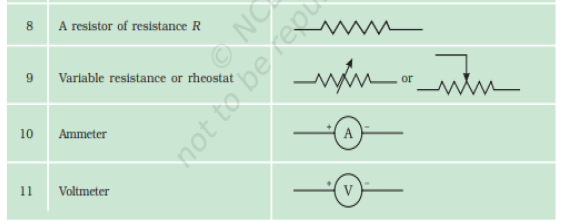# CBSE Class 10 Physics Revision Assignment Set B

Read and download free pdf of CBSE Class 10 Physics Revision Assignment Set B. Get printable school Assignments for Class 10 Physics. Standard 10 students should practise questions and answers given here for Physics in Grade 10 which will help them to strengthen their understanding of all important topics. Students should also download free pdf of Printable Worksheets for Class 10 Physics prepared as per the latest books and syllabus issued by NCERT, CBSE, KVS and do problems daily to score better marks in tests and examinations

## Assignment for Class 10 Physics

Class 10 Physics students should refer to the following printable assignment in Pdf in standard 10. This test paper with questions and answers for Grade 10 Physics will be very useful for exams and help you to score good marks

### Class 10 Physics Assignment

(ELECTRICITY)

Types of electricity
i. Static electricity
ii. Current electricity

Static Electricity: Static electricity is a type of electricity when electric charges develop but do not flow.
For example charges developed by glass rod are rubbed with silk.

Current Electricity: Current electricity is a type of electricity when the electronic charges that develop are also in motion.
For example electricity used in our homes.

Electrostatic potential
It is the work done in moving the positive charge from infinity to a certain point in an electric field of other charge.

OR The electrical potential is defined as the capability of the charged body to do work. When the body is charged, either electric electrons are supplied to it, or they are removed from it. In both the cases, the work is done. This work is stored in the body in the form of electric potential. Thus, the body can do the work by exerting a force of attraction or repulsion on the other charged particles.For example - A unit of positive charge is at a point at infinity and we try to bring it to point b that is in the electric field of some other charge, let’s say charge q2. So the work that we need to do in doing so is called electrostatic potential.

Potential difference
When we need to move the charge between two specified points, then the work done in doing so is called the potential difference. So it is defined as the work done in moving a charge from one point to another point.

OR

The electrical potential difference is defined as the amount of work done to carrying a unit charge from one point to another in an electric field. In other words, the potential difference is defined as the difference in the electric potential of the two charged bodies.Therefore;
Voltage = Work done /charge
Voltage or electric potential difference is denoted by ‘V’. Therefore;
1V=1 Joule/1 Coulomb

i.e. V= W/q (from one point to another)

The unit that is used to measure it is volt and we can define 1 volt as :1v = 1 joule 1/coulomb

So it is defined as: Potential difference is said to be 1 volt when 1J of work is done in moving 1 Coulomb of charge from one point to another. The instrument that is used to measure its magnitude is called Voltmeter.
There is an important feature that how we connect it. It is connected in parallel because it has a high resistance and also in parallel the potential difference remains constant.

Voltmeter: An apparatus to measure the potential difference or electric potential difference between two points in an electric circuit.

Electric current
As we have understood that charges do move, so here comes another physical quantity that is the rate of flow of electric charge through a conductor.
I = Q /T

The Unit that is used to measure it is ampere. 1A = 1C /1 sec

We can define 1 ampere current as
1Ampere is defined as when 1Coulomb of charge flows through wire in 1second. The ammeter is an instrument used to measure the current. Now the connection of ammeter in circuit is that it is connected in series. It has low resistance and in series, the current remains constant.

Q) How can we get continuous flow of current in a circuit?
Ans) We can get continuous flow of current in a circuit by maintaining a potential difference between the 2 ends of a conductor. We can do that by connecting it to a battery or cell because there are two terminals that are always at different potential levels.

Types of current:
Conventional                                       Electric
Flow of protons                                   Flow of electrons
More from +ve terminal to –ve             Move from – ve terminal to +ve terminal.
terminal in outer circuit.
practically not important                      Practically important

Electric circuits
A continuous conducting path between the terminals of a source of electric energy and other electrical components along which the electric current flows is called an electric circuit. Types of electric circuits:
i.Closed electric circuit
ii. Open circuitOpen electric circuit : It is the circuit in which electric contact is broken at some point such that no current flows through the components of the circuit.

Closed electric circuit : The circuit in which all the components of a circuit are joined to one another such that a continuous current flows through them is a closed electric circuit. Some electronic devices with symbols used are:

Rheostat - It is defined as the variable resistance. It is connected to change the current in the circuit without changing the voltage source like cell etc.

Galvanometer- It is a device used to measure a very small amount of current as it has very low resistance.QUESTIONS BASED ON ABOVE TOPICS:-

Question. Calculate the work done if a charge of 5 C moving across two point having potential difference equal to 15 V.
Answer. Given, potential difference (V) = 15 V
Charge (Q) = 5 C
Work done (W) =?
We know that; V=WQV=WQ
Or, W=V×QW=V×Q
Or, W=15V×5C=75JW=15V×5C=75J

Question. Calculate the work done to carry a charge of 3 C, if the potential difference between two points is 10 V.
Answer. Given, charge = 3 C
Potential difference between two points = 10V
Work done (W) =?
We know that; V=WQV=WQ
Or, W=V×QW=V×Q
Or, W=10V×3C=30J

Question. How is an ammeter connected in a circuit to measure current flowing through it?
Answer. An ammeter connected in series in a circuit to measure current flowing through it.

Question. What does an electric circuit mean?
Answer. A continuous closed path made of electric components through which an electric current flows is known as an electric circuit. A simple circuit consists of the following components: (a) Conductors
(b) Cell
(c) Switch

Question. Define the unit of current.
Answer. The unit of current is ampere. Ampere is defined by the flow of one coulomb of charge per second.

Question. Calculate the number of electrons constituting one coulomb of charge.
Answer. The value of the charge of an electron is 1.6 × 10-19 C.
According to charge quantization,
Q = nqe, where n is the number of electrons and qe is the charge of an electron.
Substituting the values in the above equation, the number of electrons in a coulomb of charge can be calculated as follows:Therefore, the number of electrons constituting one coulomb of charge is 25 × 1018.

Question. Name a device that helps to maintain a potential difference across a conductor.
Answer. Battery consisting of one or more electric cells is one of the devices that help to maintain a potential difference across a conductor.

Question. What is meant by saying that the potential difference between two points is 1 V?
Answer. When 1 J of work is done to move a charge of 1 C from one point to another, it is said that the potential difference between two points is 1 V.

Question. How much energy is given to each coulomb of charge passing through a 6 V battery?
Answer. We know that the potential difference between two points is given by the equation,
V = W/Q, where,
W is the work done in moving the charge from one point to another
Q is the charge
From the above equation,
we can find the energy given to each coulomb as follows:
W = V × Q
Substituting the values in the equation,
we get W = 6V × 1C = 6 J
Hence, 6 J of energy is given to each coulomb of charge passing through a 6 V of battery.

Question. Define charge?
Answer. Electric charge is the physical property of matter that causes it to experience a force when placed in an electromagnetic field. There are two types of electric charge: positive and negative

Question. Write the properties of charge.
Answer. Electrically charged objects have several important characteristics:
1. Like charges repel one another; that is, positive repels positive and negative repels negative.
2. Unlike charges attract each another; that is, positive attracts negative.
3. Charge is conserved.
4. Charge is quantized.

Question. What are five examples of conductors?
• silver.
• copper.
• gold.
• aluminium.
• iron.
• steel. Etc.

Please click the below link to access CBSE Class 10 Physics Revision Assignment Set B

 CBSE Class 10 Science Chemical Reactions and Equations Assignment
 CBSE Class 10 Science Acids Bases and Salts Assignment Set A CBSE Class 10 Science Acids Bases and Salts Assignment Set B CBSE Class 10 Science Acids Bases and Salts Assignment Set C
 CBSE Class 10 Science Metals and Non Metals Assignment Set A CBSE Class 10 Science Metals and Non Metals Assignment Set B
 CBSE Class 10 Science Carbon and its compounds Assignment Set A CBSE Class 10 Science Carbon and its compounds Assignment Set B CBSE Class 10 Science Carbon and its compounds Assignment Set C CBSE Class 10 Science Carbon and its compounds Assignment Set D CBSE Class 10 Science Carbon and its compounds Assignment Set E
 CBSE Class 10 Science Periodic Classification Of Elements Assignment Set A CBSE Class 10 Science Periodic Classification Of Elements Assignment Set B CBSE Class 10 Science Periodic Classification Of Elements Assignment Set C
 CBSE Class 10 Science Biology Life Process Respiration Assignment CBSE Class 10 Science Biology Nutrition Assignment CBSE Class 10 Science Biology Respiration Assignment CBSE Class 10 Science Biology Transport Enrichment Assignment CBSE Class 10 Science Biology Transportation Assignment CBSE Class 10 Science Life Processes Assignment Set A CBSE Class 10 Science Life Processes Assignment Set B CBSE Class 10 Science Life Processes Assignment Set C
 CBSE Class 10 Science Control and Coordination Assignment Set A CBSE Class 10 Science Control and Coordination Assignment Set B CBSE Class 10 Science Control and Coordination Assignment Set C CBSE Class 10 Science Control and Coordination Assignment Set D
 CBSE Class 10 Science How do Organisms Reproduce Assignment Set A CBSE Class 10 Science How do Organisms Reproduce Assignment Set B
 CBSE Class 10 Science Heredity and Evolution Assignment Set A CBSE Class 10 Science Heredity and Evolution Assignment Set B CBSE Class 10 Science Heredity and Evolution Assignment Set C
 CBSE Class 10 Science Light Reflection and Refraction Assignment Set A CBSE Class 10 Science Light Reflection and Refraction Assignment Set B CBSE Class 10 Science Light Reflection and Refraction Assignment Set C CBSE Class 10 Science Light Reflection and Refraction Assignment Set D CBSE Class 10 Science Light Reflection and Refraction Assignment Set E CBSE Class 10 Science Light Reflection and Refraction Assignment Set F
 CBSE Class 10 Science Human Eye and Colorful World Assignment Set A CBSE Class 10 Science Human Eye and Colorful World Assignment Set B CBSE Class 10 Science Human Eye and Colorful World Assignment Set C
 CBSE Class 10 Physics Electricity Assignment Set A CBSE Class 10 Physics Electricity Assignment Set B CBSE Class 10 Physics Electricity Assignment Set C CBSE Class 10 Science Electricity Assignment Set A CBSE Class 10 Science Electricity Assignment Set B CBSE Class 10 Science Electricity Assignment Set C
 CBSE Class 10 Science Magnetic Effects of Electric Current Assignment Set A CBSE Class 10 Science Magnetic Effects of Electric Current Assignment Set B CBSE Class 10 Science Magnetic Effects of Electric Current Assignment Set C CBSE Class 10 Science Magnetic Effects of Electric Current Assignment Set D
 CBSE Class 10 Science Sources of Energy Assignment Set A CBSE Class 10 Science Sources of Energy Assignment Set B CBSE Class 10 Science Sources of Energy Assignment Set C CBSE Class 10 Science Sources of Energy Assignment Set D
 CBSE Class 10 Science Our Environment Assignment Set A CBSE Class 10 Science Our Environment Assignment Set B CBSE Class 10 Science Our Environment Assignment Set C
 CBSE Class 10 Science Sustainable Management of Natural Resources Assignment Set A CBSE Class 10 Science Sustainable Management of Natural Resources Assignment Set B CBSE Class 10 Science Sustainable Management of Natural Resources Assignment Set C
 CBSE Class 10 Physics Revision Assignment Set A CBSE Class 10 Physics Revision Assignment Set B CBSE Class 10 Physics Revision Assignment Set C CBSE Class 10 Physics Revision Assignment Set D CBSE Class 10 Physics Revision Assignment Set E CBSE Class 10 Physics Revision Assignment Set F CBSE Class 10 Physics Revision Assignment Set G CBSE Class 10 Physics Revision Assignment Set H
 CBSE Class 10 Science Assignment CBSE Class 10 Science Assignments Collection CBSE Class 10 Science Energy Crossword Puzzle Assignment
 CBSE Class 10 Physics Question Bank CBSE Class 10 Physics Tungsten Assignment
 CBSE Class 10 Science Chemistry Cleaning Capacity of Soap Assignment CBSE Class 10 Science Chemistry Determination of pH Assignment CBSE Class 10 Science Chemistry Different Types of Chemical Reactions Assignment CBSE Class 10 Science Chemistry Displacement Reaction and Reactivity Series Assignment CBSE Class 10 Science Chemistry Properties of Acetic Acid Assignment CBSE Class 10 Science Chemistry Saponification Preparation of Soap Assignment CBSE Class 10 Science Chemistry Study of properties of HCI Acid and NaOH Assignment

Tags